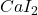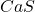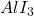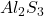Write the empirical formula of at least four binary ionic compounds that could be formed from the following ions: Ca^2 , Al^3 ,I^-,S^2-

Question

Write the empirical formula of at least four binary ionic compounds that could be formed from the following ions: Ca^2 , Al^3 ,I^-,S^2-

in progress 0
6 months 2021-07-14T02:38:40+00:00 1 Answers 40 views 0

Write the empirical formula of at least four binary ionic compounds that could be formed from the following ions: Ca^2 , Al^3 ,I^-,S^2-

Explanation:

1)Formula of calcium iodide:

The compound of Ca^2+ and I^-1:2) Formula of calcium sulfide:3) Formula of aluminum iodide:4) Formul aluminum sulfide:These are the four ionic compounds that are formed.